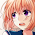## 20 Nov 2012

This VBA procedure (1) Searches words ("Str1", "Str2",..) and adds commentboxes to it and (2) changes the initials used in the box:

```Sub CommentBox()

Dim range As range
Dim i As Long
Dim TargetList
Dim cm As Comment

TargetList = Array("Str1", "Str2")

For i = 0 To UBound(TargetList)

Set range = ActiveDocument.range

With range.Find
.Text = TargetList(i)
.Format = True
.MatchCase = False
.MatchWholeWord = True
.MatchWildcards = False
.MatchSoundsLike = False
.MatchAllWordForms = False

Do While .Execute(Forward:=True) = True

cm.Author = "InternalName"
cm.Initial = "Initials"

Loop

End With

Next

With ActiveDocument.Styles("Kommentartext").Font
.Size = 12
.Bold = True
.Italic = True
.Color = wdColorBlue
End With

End Sub

```

#### 1 comment :

1.Nice Post ,I Like This Post ,thanks for sharing